# The cylinder shown is of weight W and radius r. Express in terms XV and r the magnitude of the largest couple M that can be applied to the cylinder if it is not to rotate, assuming the coefficient of static friction to be (a) zero at A and 0.30 at B, (b) 0.25 at A and 0.30 at B.

Question-AnswerCategory: Engineering MechanicsThe cylinder shown is of weight W and radius r. Express in terms XV and r the magnitude of the largest couple M that can be applied to the cylinder if it is not to rotate, assuming the coefficient of static friction to be (a) zero at A and 0.30 at B, (b) 0.25 at A and 0.30 at B.

The cylinder shown is of weight W and radius r. Express in terms XV and r the magnitude of the largest couple M that can be applied to the cylinder if it is not to rotate, assuming the coefficient of static friction to be (a) zero at A and 0.30 at B, (b) 0.25 at A and 0.30 at B.Step: 1

Let us draw the free body diagram of the cylinder.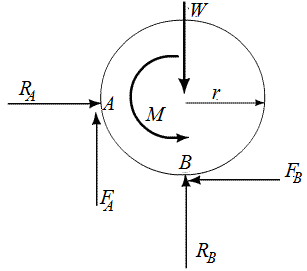Step: 2

a)
Given
The coefficient of static friction at A is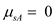The coefficient of static friction at B is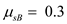Step: 3

The frictional force acting at A,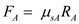The frictional force acting at B,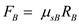So,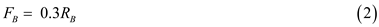Step: 4

Assume that the force in the positive x-direction is positive.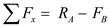Take the condition of equilibrium,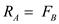Substitute the value of FB from equation (2) and solve.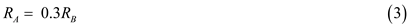Step: 5

Assume that the force in the positive y-direction is positive.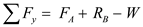Take the condition of equilibrium,Substitute the value of FA from equation (1) and solve.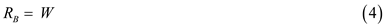Step: 6

Assume that the moment in the anticlockwise direction is positive.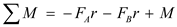Take the condition for equilibrium,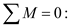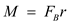Substitute the value of FB from equation (2) and solve.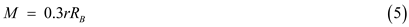Substitute the value of RB from equation (4) and solve.Therefore, the magnitude of the largest couple that can be applied to the cylinder if it is not to rotate is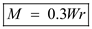Step: 7

b)
Given
The coefficient of static friction at A isThe coefficient of static friction at B isStep: 8

The frictional force acting at A,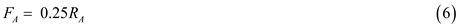The frictional force acting at B,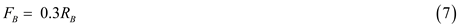Take the condition of equilibrium,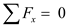and apply the condition of equilibrium. Assume that the force in the positive x-direction is positive.Substitute the value of FB from equation (7) and solve.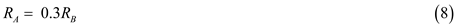Assume that the force in the positive y-direction is positive.Take the condition of equilibrium,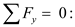Substitute the value of FA from equation (6) and solve.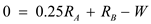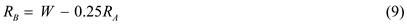Step: 9

Assume that the moment in the anticlockwise direction is positive.Take the condition for equilibrium,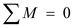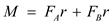Substitute the value of FA and FB from equation (6) and equation (7) and solve.Step: 10

Substitute the value of RA from equation (8) in equation (9) and solve.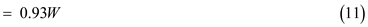Substitute the value of RB from equation (11) in equation (8) and solve.Step: 11

Substitute the value of RB and RA from equation (11) and equation (12) in equation (10) and solve.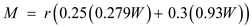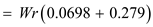.
Therefore, the magnitude of the largest couple that can be applied to the cylinder if it is not to rotate is